### Geometry

1. The sum of the interior angles of a polygon is 1620°. The number of sides of the polygon are

2. A cyclic parallelogram having unequal adjacent sides is necessarily a :

3. If the angles of a triangle are in the ratio 5 : 3 : 2, then the triangle could be :

4. If one of the diagonals of a rhombus is equal to its side, then the diagonals of the rhombus are in the ratio:

5. In the given figure, ∠ ABC and ∠ DEF are two angles such that BA ⊥ ED and EF ⊥ BC, then find value of ∠ ABC + ∠ DEF.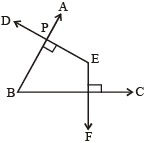6. In the given figure given below, E is the mid-point of AB and F is the midpoint of AD. if the area of FAEC is 13, what is the area of ABCD?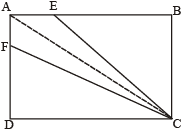7. Give that segment AB and CD are parallel, if lines ℓ, m and n intersect at point O. Find the ratio of θ to ∠ODS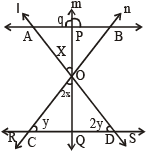8. AB ⊥ BC and BD ⊥ AC. CE bisects the angle C. ∠A = 30°. Then, what is ∠CED?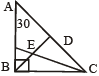9. In triangle ABC, angle B is a right angle. If (AC) is 6 cm, and D is the mid – point of side AC. The length of BD is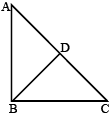10. ABCD is a square of area 4, which is divided into four non overlapping triangles as shown in the fig. Then the sum of the perimeters of the triangles is11. The sides of a quadrilateral are extended to make the angles as shown below :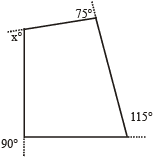What is the value of x?

12. Instead of walking along two adjacent sides of a rectangular field, a boy took a short cut along the diagonal and saved a distance equal to half the longer side. Then the ratio of the shorter side to the longer side is

13. If two parallel lines are cut by two distinct transversals, then the quadrilateral formed by these four lines will always be a :

14. In the following figure, find ∠ADC.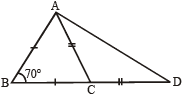15. In a triangle ABC, the internal bisector of the angle A meets BC at D. If AB = 4, AC = 3 and ∠A = 60°, then the length of AD is

16. In the figure AG = 9, AB = 12, AH = 6, Find HC.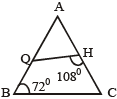17. In ∆ABC, DE || BC and AD/DB = 3/5. If AC = 5.6 cm, find AE.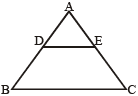18. In the given fig. AB || QR, find the length of PB.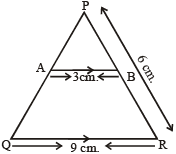19. In a triangle ABC, the lengths of the sides AB, AC and BC are 3, 5 and 6 cm, respectively. If a point D on BC is drawn such that the line AD bisects the angle A internally, then what is the length of BD?

20. The number of tangents that can be drawn to two non-intersecting circles is :